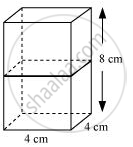# 2 cubes each of volume 64 cm^3 are joined end to end. Find the surface area of the resulting cuboid. - Mathematics

2 cubes each of volume 64 cm3 are joined end to end. Find the surface area of the resulting cuboid.

#### Solution

Given that,

Volume of cubes = 64 cm3

(Edge) 3 = 64

Edge = 4 cmIf cubes are joined end to end, the dimensions of the resulting cuboid will be 4 cm, 4 cm, 8 cm.

∴Surface area of cuboids = 2(lb+bh+lh)

= 2(4x4+4x8+4x8)

=2(16+32+32)

2(16+64)

=2x80 = 160 cm2

Is there an error in this question or solution?
Chapter 13: Surface Areas and Volumes - Exercise 13.1 [Page 244]

#### APPEARS IN

NCERT Class 10 Maths
Chapter 13 Surface Areas and Volumes
Exercise 13.1 | Q 1 | Page 244

Share